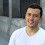## Tuesday, March 24, 2020

Published March 24, 2020 by with 3 comments

# How to Work With Log Scales

Log scales come up often and can be confusing. Here's a quick guide.

#### How to read a log scale

The easiest way to understand a log scale is to understand that two sets of numbers separated by the same distance will have the same ratio when you divide them. For example, 1 and 2 will be separated by the same distance as 10 and 20 on a log scale since 2 / 1 = 2, and 20 / 10 = 2.

You typically have both major and minor grid lines drawn. The plots below are a common way to represent it with both grid lines drawn and with major ticks labelled. When done this way, the minor ticks are 2, 3, 4, 5, 6, 7, 8, and 9 times the nearest, lower major tick. That is, the minor ticks between 100 and 1,000 are 200, 300, 400, 500, 600, 700, 800, and 900. Notice that they are not evenly spaced. As noted above, equal ratios get equal spacing, so the distance between ticks 200 and 100 is the same as between 400 and 200 and 800 and 400, and those are all spaced further apart than 900 and 600.

As an example, consider the following plot:

How long does it take for the value to increase by 10x? That's kind of hard to tell. You could try to read off a bunch of points but it grows so rapidly that it's hard to do accurately. You could try some curve fits and derive the value from the equation. Overally it's not too obvious though.

Now consider the same plot with a log scale for the vertical axis:

That question is much easier now. The value clearly goes from 100 to 1,000 between 1960 and 1980, and from 1,000 to 10,000 between 1980 and 2000. It takes 20 years to increase by 10x.

For another example, how much did the value drop in the dip at 1960 here?

No idea. It's extremely difficult to tell how much was lost there.

Same plot with a log scale for the vertical axis is much clearer. It clearly drops from 400 to 200 which is a loss of 50%.

One more...imagine this curve is the growth of something like the stock market. It has two crashes seen by the two dips here. How do the crashes compare in % loss?

Given how hard it is to read the size of the 1960 dip, I can't really say quickly. It sure looks like the 1990 one was much larger though. Now look at it with a log scale for the vertical axis:

The dips were identical. both were a ~50% drop. It's easier to read something like that on with a log scale.

#### When should you use a log scale?

In general, anytime something grows by a fixed percentage of present value, a log scale is probably a good idea. If you say something like 'we expect the company to grow 5% year over year', that company's value should go on a log scale. Another way of saying it is if the change in a value depends on the current value, a log scale might make sense.

Here are some examples of situations that make sense for it:
• economic growth like the S&P 500 vs time or the US gdp vs time (each year it grows by 3% or whatever)
• spread of a contagious virus in its early stages (each infected person spreads it to 3 more people and they spread it to 3 more people...)
• population growth in its early stages (each couple has 3 kids, and their kids each have 3 kids...)
Here are some examples of situations that don't make sense for it:
• points in a basketball game vs time left in the game (points are roughly equally likely throughout the game though there is often a spike at the end with free throws)
• distance travelled when moving at a steady velocity

There's a trick that's commonly used that works roughly the same way, and that's to convert your dataset to a logarithmic unit. The most common example is the decibel when used for sound levels. A motorcycle passing by is often roughly 90 decibels. How loud are two motorcycles passing by? 180 decibels right? No...that would be louder than a space shuttle launching (people have to be miles away to avoid going deaf from those).

You don't add decibels that way. Doubling the actual intensity of the source adds 3 decibels. Something that's 10 decibels louder than another thing is perceived by humans to be roughly twice as loud.

1.2.3.